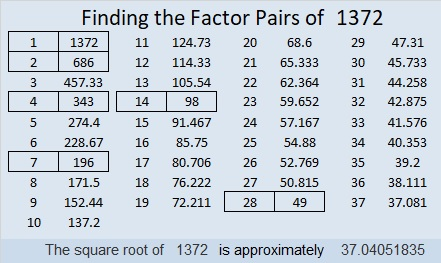# How Much of My \$1540 Will The Taxman Get?

### The Taxman Game

You might think that the picture below is just the numbers from 1 to 55 arranged in a 5×11 rectangle.

It’s really PLAY MONEY, one bill in each denomination from 1 to 55. If you add up the total value of all this play money, you will get 1540 because the sum of the numbers from 1 to 55 is 1540.Most of that 1540 in play money can be yours to keep if you outsmart THE TAXMAN.

You can take any bill that you want, but The Taxman has to get paid every time you do. The Taxman will get ALL the available factors of the bill you take. If there isn’t at least one bill on hand that is a factor of your chosen bill, then you can’t take that bill. When none of the bills left are factors of any of the other bills that are left, The Taxman gets ALL the remaining bills. The value of those bills could add up quickly, and that’s how The Taxman might outsmart you.

You can print this excel file to play the Taxman game: Taxman & 1537-1544 It has all the play money bills from 1 to 100, and all the factors are typed on the top of the bills!

The factors at the top of each bill make it possible to play the game even if you don’t know how to multiply or divide yet. My granddaughter learned to play the game sometime around her eighth birthday. Before tackling 55 bills, she learned what to do for a smaller number of bills. I told her:
If 1 is the only bill, The Taxman wins.
If 1, 2 are the only bills, you take the 2, The Taxman gets the 1, and you win.
If 1, 2, 3 are the only bills, the best you can do is a draw (1 + 2 = 3),
But if there are at least four bills, it is possible to win every time.

She played the game over and over adding one more bill to the game each time she played. She enjoyed it very much and played it over 20 times until bedtime required her to stop. Each time she played we talked about how she would arrange the cards in a rectangle. For example, if she used cards 1 – 6, she would look at the factors of 6 and decide on a 2 × 3 rectangle or a 1 × 6 rectangle.

Knowing I wanted to write this post about the 55th triangular number (1540), I had her jump to using 55 bills the next day, but I helped her with it a little. We didn’t want to just win the game, we wanted to make it so The Taxman would get as little money as possible. Here is the final order of what we took and what The Taxman got.Laying it out like that made it easy to have do-overs when needed.

### A Way to Keep Score When Playing Taxman:

When it was time to score the game, instead of adding up all the numbers to see if we took more than The Taxman, we found smaller sums that equaled as many of our choices as we could as illustrated below. For example,  47 + 6 = 53, so there was no need to add 47 + 6 to The Taxman’s total or to add 53 to ours.I found it enjoyable finding all those sums, but it isn’t necessarily easy for a child to do. After arranging all those sums on the table, we were able to see that we scored
35 + 39 + 38 + 12 + 30 + 50 + 24 + 48 + 42 = 318 more than The Taxman did.

We can use algebra to figure out exactly how much of our 1540 in play money we get to keep.
If The Taxman got X of the play money, we kept X + 318 of it, and
X + X + 318 = 1540,
2X + 318 = 1540,
2X + 318 – 318 = 1540 – 318,
2X = 1222,
X = 611, that’s what The Taxman got.
We kept 611 + 318 = 929 of our play money.

What percentage of the play money did The Taxman get?
611/1540 ≈ 0.40 which is 40%. Wow, that’s a lot!

### Factors of 1540:

• 1540 is a composite number.
• Prime factorization: 1540 = 2 × 2 × 5 × 7 × 11, which can be written 1540 = 2² × 5 × 7 × 11
• 1540 has at least one exponent greater than 1 in its prime factorization so √1540 can be simplified. Taking the factor pair from the factor pair table below with the largest square number factor, we get √1540 = (√4)(√385) = 2√385
• The exponents in the prime factorization are 2, 1, 1, and 1. Adding one to each exponent and multiplying we get (2 + 1)(1 + 1)(1 + 1)(1 + 1) = 3 × 2 × 2 × 2 = 24. Therefore 1540 has exactly 24 factors.
• The factors of 1540 are outlined with their factor pair partners in the graphic below.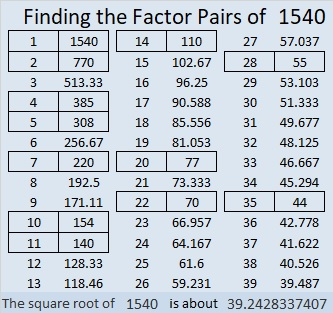### Factor Cake for 1540:

1540 has lots of prime factors with 11 being the greatest so it makes a lovely factor cake:### What Kind of Shape Is 1540 in?

I know that 1540 = 55×56÷2 so, 1540 is the 55th triangular number. Guess what? Since 55 is also a triangular number (55 = 10×11÷2), we could have arranged the play money bills in a triangle like this instead of a 5 × 11 rectangle.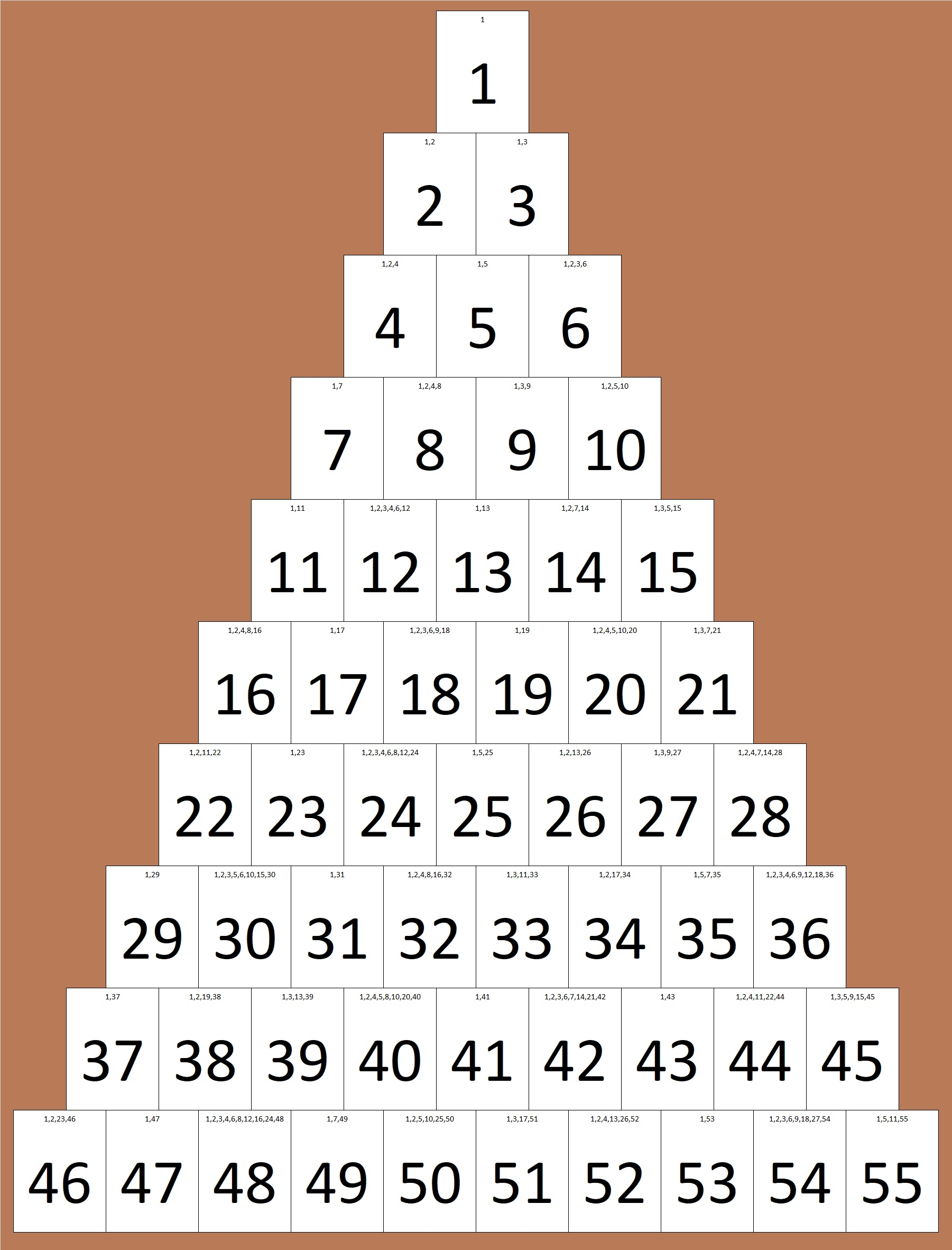1540 is not only the 55th triangular number, but it is also the sum of the first 20 triangular numbers:

1+3+6+10+15+21+28+36+45+55+66+78+91+105+120+136+153+171+190+210 = 1540.

If you stack those twenty triangular numbers from smallest to biggest, you will get something shaped like a tetrahedron. That’s why we say that 1540 is the 20th tetrahedral number. We can also use a formula for the 20th tetrahedral number:
20(21)(22)/6 = 1540.

1540 is also the 28th hexagonal number. (All hexagonal numbers are also triangular numbers. That’s why it’s the 2(28)-1 = 55th triangular number.) It is the 28th hexagonal number because 2(28²) – 28 = 1540.1540 is the 20th decagonal number because 4(20²) – 3(20) = 1540.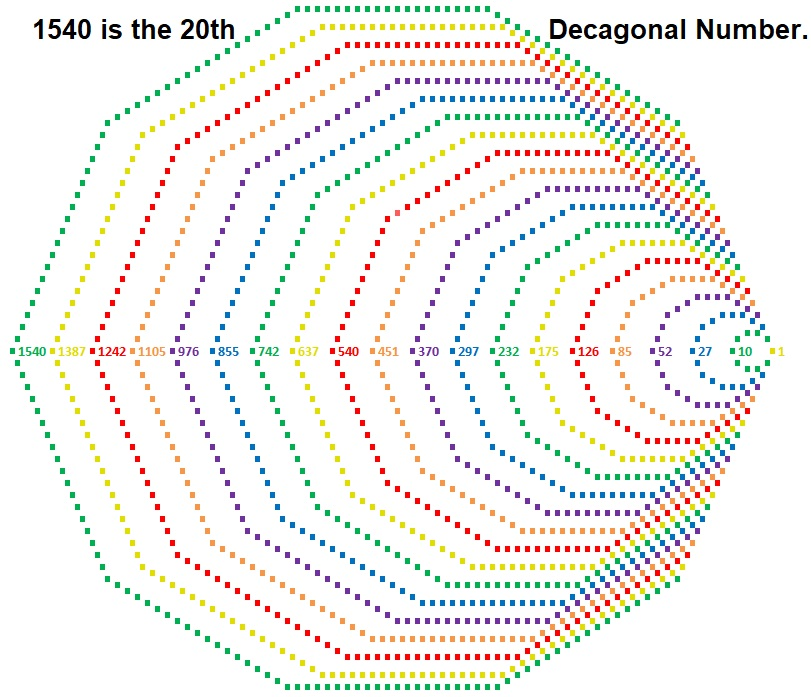I didn’t make a visual, but 1540 is also the19th centered nonagonal number.
That’s because
3 × 19 = 57 and (57 – 2)(57 – 1)/2 = 1540.

1540 is also the hypotenuse of a Pythagorean triple triangle:
924-1232-1540 which is (3-4-5) times 308.

1540 is quite the shape-shifter number, isn’t it!

# 1386 What You Need to Know About the Multiplication Game

I recently wrote about Hooda Math’s Multiplication Game. There’s a couple of things I didn’t tell you in that post.

First of all, you don’t have to use a computer to play the game. (However, using one the first time you play will help you understand how to play). You can print a game board to play. I’ve created a game board below that you could use. Each player can use different items such as beads, pennies, nickels, and dimes as markers to mark the factors used and to claim the resulting products on the game board.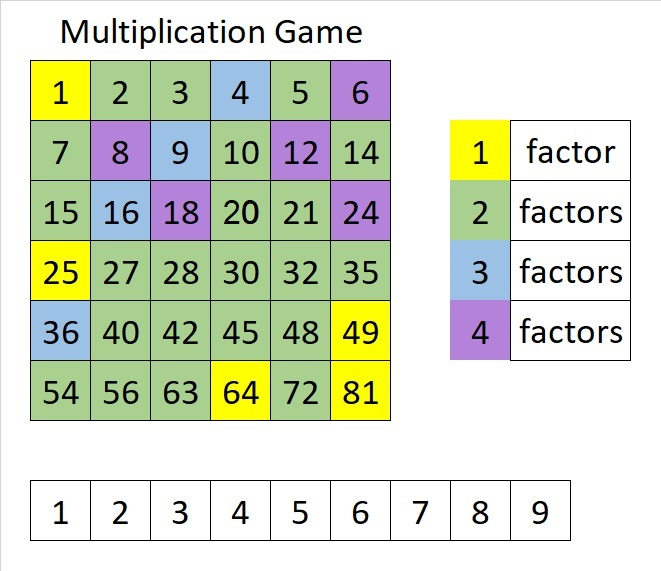The second thing you should know is that getting four squares in a row, horizontally, vertically or diagonally is NOT equally likely every place on the board. If one particular number is all you need to get a win, you are less likely to get that number if it only has one factor (like the numbers marked in yellow have). As far as this game is concerned, the products have the number of factors that I’ve indicated, even though in reality most of them have more than that.

You can’t win unless your opponent gives you one of the factors you need to claim that winning space. If 4 of the 9 possible factors will get it for you, the odds are much better your opponent will give you what you need than if only 1 of the 9 possible factors will do it.

If you know which numbers have four possible factors, you may have an advantage over someone who thinks this game is really just a variation of tic-tac-toe. Of course, those products with four factors could also make you more likely to get blocked as well! And if you use my colorful game board, your opponent will know just as much as you do about how many ways they can get each square.

### 1386 Factor Cake:

Since the biggest prime factor of 1386 is 11, it makes an especially festive factor cake!### Factors of 1386:

Now I’ll share some information about the number 1386:

• 1386 is a composite number.
• Prime factorization: 1386 = 2 × 3 × 3 × 7 × 11, which can be written 1386 = 2 × 3² × 7 × 11
• 1386 has at least one exponent greater than 1 in its prime factorization so √1386 can be simplified. Taking the factor pair from the factor pair table below with the largest square number factor, we get √1386 = (√9)(√154) = 3√154
• The exponents in the prime factorization are 1, 2, 1, and 1. Adding one to each exponent and multiplying we get (1 + 1)(2 + 1)(1 + 1)(1 + 1) = 2 × 3 × 2 × 2 = 24. Therefore 1386 has exactly 24 factors.
• The factors of 1386 are outlined with their factor pair partners in the graphic below.### Sum-Difference Puzzle:

1386 has twelve factor pairs. One of the factor pairs adds up to 85, and a different one subtracts to 85. If you can identify those factor pairs, then you can solve this puzzle!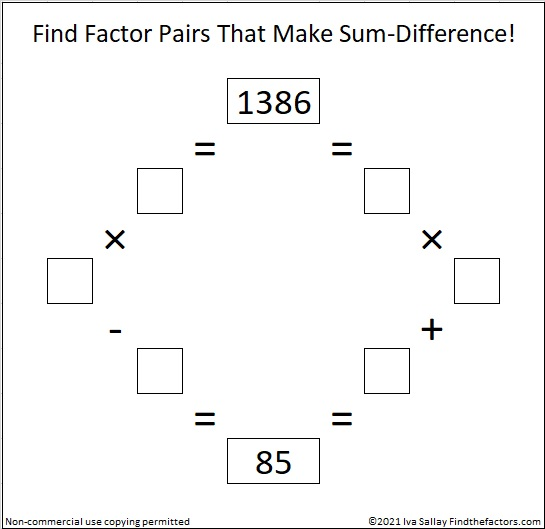### One More Fact about the Number 1386:

OEIS.org also noted that 1 + 3⁴ + 8 + 6⁴ = 1386.

# 1381 Hooda Math’s Multiplication Game

The school year is almost over, and class periods were only twenty-five minutes long today. I went online looking for math games that would benefit my students and I found a winner with Hooda Math’s Multiplication Game.If you count the multiplication facts in a 9×9 multiplication table, you will see 99 facts, but many of the products are duplicated in the table. Every yellow square below is also in white elsewhere in the table:There are actually only 36 unique products in the multiplication table above. Hooda Math has cleverly arranged those 36 products in a 6×6 grid that becomes the game board. In this two-person game, students take turns moving one of two arrows to a number from 1 to 9 at the bottom of the screen and claiming the square that contains the product of the numbers. The catch is that players must keep one of the numbers chosen by the previous player and cannot claim a product that has already been claimed by either player. (Player 1 cannot score on his first turn.) One student is green and the other is purple and the first to claim four squares in a row is the winner. The rules on the website are VERY short and simple.

Students played this game today. I played it as well. Sometimes I won, and sometimes I lost, but the losses are more interesting than the wins:

In one game, my opponent took the square that I needed to get four in a row vertically for the win. All she was trying to do was block me from winning, however, when she took that square, the game declared her the winner. We were puzzled why she was the winner until she figured out that making that move gave her four in a row diagonally. That’s when we found out players can win by getting four in a row diagonally as well as vertically or horizontally.

In another game, I had two possible moves that would have made me be the winner. I just needed my opponent to choose a 1, 6, or 8 as their other factor, and I would win with 1 × 1 = 1 or 6 × 8 = 48. Unfortunately, he knew to beware of the numbers that would make me win. One of the arrows was pointing to 5, and he made the other arrow point to 5. By now there were no other products left on the board that were divisible by 5, so I couldn’t win because I couldn’t move either of the arrows.

That’s how I didn’t win the game either of those times, but I had a lot of fun anyway, and you will, too!

This is my 1381st post. Here’s some information about that number:

• 1381 is a prime number.
• Prime factorization: 1381 is prime.
• 1381 has no exponents greater than 1 in its prime factorization, so √1381 cannot be simplified.
• The exponent in the prime factorization is 1. Adding one to that exponent we get (1 + 1) = 2. Therefore 1381 has exactly 2 factors.
• The factors of 1381 are outlined with their factor pair partners in the graphic below.How do we know that 1381 is a prime number? If 1381 were not a prime number, then it would be divisible by at least one prime number less than or equal to √1381. Since 1381 cannot be divided evenly by 2, 3, 5, 7, 11, 13, 17, 19, 23, 29, 31, or 37, we know that 1381 is a prime number.

1381 is the 24th Centered Pentagonal Number because
5(23)(24)/2 + 1 = 1381. In formula means that it is one more than five times the 23rd triangular number, as I’ve illustrated below:1381 is the sum of two squares:
34² +15² = 1381

1381 is the hypotenuse of a Pythagorean triple:
931-1020-1381 calculated from 34² -15², 2(34)(15), 34² +15²

Here’s another way we know that 1381 is a prime number: Since its last two digits divided by 4 leave a remainder of 1, and 34² + 15² = 1381 with 34 and 15 having no common prime factors, 1381 will be prime unless it is divisible by a prime number Pythagorean triple hypotenuse less than or equal to √1381. Since 1381 is not divisible by 5, 13, 17,  29, or 37, we know that 1381 is a prime number.

# 1372 Yahtzee How-Many-Rolls Variation

Today on the spot I made up a quick variation of Yahtzee, and one of my students played it with me.

The object of the game was to get all five dice to show the same number of dots at the same time, but instead of only being allowed to have up to three rolls, we took as many rolls as need. To take a turn, one of us would roll the dice then look to see if any of the dice were the same. Any die that didn’t match would be included in an additional roll until it did match. We counted each roll we took and got one point for each roll. The lowest score would determine the winner. The student and I played four rounds. He was elated because he won EVERY round so, of course, he was the overall winner, too.Usually, when we play a game together the scores are much closer. Sometimes I win, sometimes he wins. Today I couldn’t believe my bad luck! Sometimes none of the dice matched after my first roll. And what about my student’s very good luck getting five of a kind in just one roll? I’m sure some good probability discussions could result from this game.

Our data might suggest that 9 rolls is the most that a person could get, but I rolled the dice for the picture included in this post, and it took me 19 rolls to get that Yahtzee! And I actually had four 4’s after just 6 rolls before I took those last 13 rolls.

You never know for sure what will happen when it comes to games of chance. If you study probability, you can have a good idea about what is most likely to happen, but you cannot guarantee it will happen. If we had taken the time to play more rounds, maybe my student would have needed 10 or more rolls to get at least one of his Yahtzees, and the game would have been more competitive. (At least, that was what I was thinking before he rolled on rounds 3 and 4.)

I’d like to encourage you to try playing this game, too. I thought it was a lot of fun even though I lost miserably.

Now I’ll write a little bit about the number 1372:

• 1372 is a composite number.
• Prime factorization: 1372 = 2 × 2 × 7 × 7 × 7 which can be written 1372 = 2² × 7³
• 1372 has at least one exponent greater than 1 in its prime factorization so √1372 can be simplified. Taking the factor pair from the factor pair table below with the largest square number factor, we get √1372 = (√196)(√7) = 14√7
• The exponents in the prime factorization are 2 and 3. Adding one to each exponent and multiplying we get (2 + 1)(3 + 1) = 3 × 4 = 12. Therefore 1372 has exactly 12 factors.
• The factors of 1372 are outlined with their factor pair partners in the graphic below.